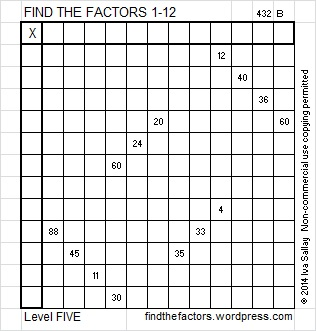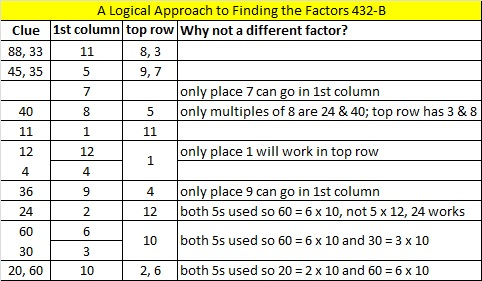# 205 and Level 5

• 205 is a composite number.
• Prime factorization: 205 = 5 x 41
• The exponents in the prime factorization are 1 and 1. Adding one to each and multiplying we get (1 + 1)(1 + 1) = 2 x 2 = 4. Therefore 205 has exactly 4 factors.
• Factors of 205: 1, 5, 41, 205
• Factor pairs: 205 = 1 x 205 or 5 x 41
• 205 has no square factors that allow its square root to be simplified. √205 ≈ 14.317821——————————————————————Excel file of puzzles and previous week’s factor solutions: 12 Factors 2014-08-11